×

Search anything:

# malloc vs calloc vs realloc

#### Software Engineering C Programming C++ memory allocation dynamic memoryGet this book -> Problems on Array: For Interviews and Competitive Programming

We take a deep look into the 3 memory allocation techniques in C/ C++ namely malloc, calloc and realloc and explore the difference.

Ever came across a problem where you are not aware about how much memory you need? Here is the solution dynamic memory allocations. This way of implementation help us to allocate memory during run time and hence you are not required to pre-define memory spaces. An example of a data structure using this concept is linked list.

C provides some functions to achieve these tasks. There are 3 library functions provided by C defined under <stdlib.h> header file to implement dynamic memory allocation in C programming. They are:

1. malloc()
2. calloc()
3. realloc()

### malloc():

Key points:

• It stand for memory allocations
• This method is used to dynamically allocate a single large block of memory with the requied size.
• It returns a pointer of type void which can be casted into a pointer of any form.

Syntax:

``````mp = (cast_type*) malloc(byte_size);
``````

Where :

• mp : pointer mp holds the address of the first byte in the allocated memory.
• cast_type : It determines data type of memory allocated.
• byte_size : It help us to determine byte of memory allocated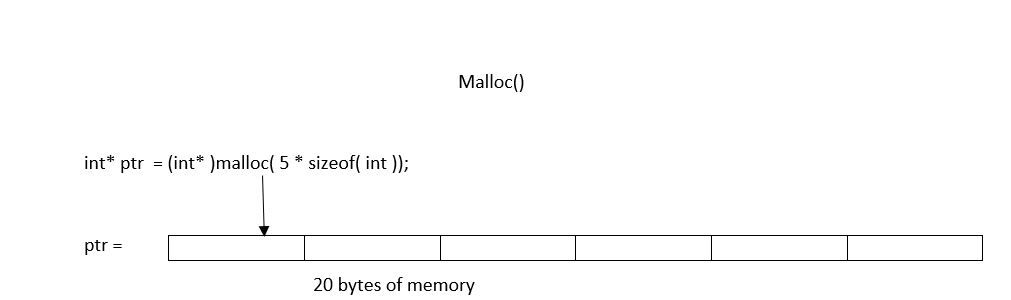Example :

``````mp = (char*) malloc(100 * sizeof(char));
``````

Since the size of char is 1 bytes this statement will allocate 100 bytes of memory.
The pointer mp holds the address of the first byte in the allocated memory.

### Pseudocode

``````#include <stdio.h>
#include <stdlib.h>

int main()
{

// This pointer will hold the
// base address of the block created
int* ptr;
int n, i, sum = 0;

// Get the number of elements for the array

printf("Number of elements in given array : \n");
scanf("%d",&n);

// Dynamically allocate memory using malloc()
ptr = (int*)malloc(n * sizeof(int));

// Check if the memory has been successfully
// allocated by malloc or not
if (ptr == NULL) {
printf("Memory not allocated.\n");
exit(0);
}
else {

// Memory has been successfully allocated
printf("Memory successfully allocated using malloc.\n");
printf("\nEnter elements in array");

// Get the elements of the array
for (i = 0; i < n; ++i) {
scanf("\n%d",&ptr[i]);
}

// Print the elements of the array
printf("The elements of the array are: ");
for (i = 0; i < n; ++i) {
printf("%d, ", ptr[i]);
}
}

return 0;
}
``````

Output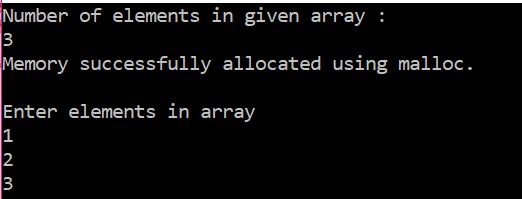### When to use malloc()

• Use malloc when you need to allocate objects that must exist beyond the lifetime of execution of the current block.

### calloc():

Key points:

• It stands for contiguous allocation.
• Similar to malloc() but in this method we also specify number of blocks of memory to be allocated.
• Each block intialized by value 0.

Syntax :

``````cp = (cast_type*)calloc(n, element_size);
``````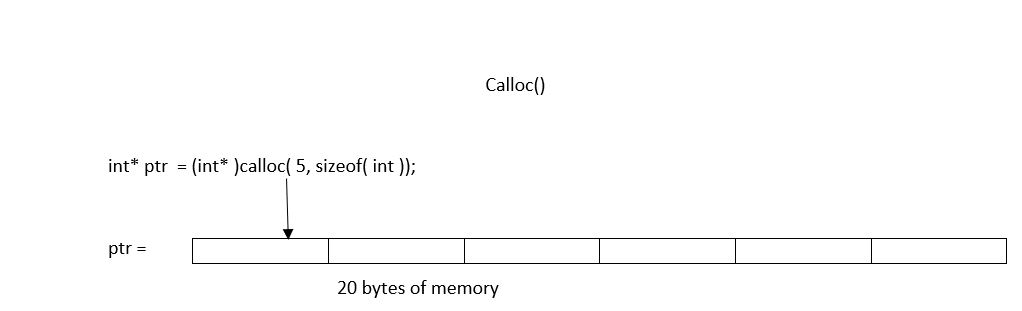Example :

``````cp= (int*) calloc(100, sizeof(int));
``````

This statement allocates contiguous space in memory for 100 elements each with the size of int.

### Pseudocode

``````#include <stdio.h>
#include <stdlib.h>

int main()
{

// This pointer will hold the
// base address of the block created
int* ptr;
int n, i, sum = 0;

// Get the number of elements for the array

printf("Enter number of elements: \n");
scanf("%d",&n);

// Dynamically allocate memory using calloc()
ptr = (int*)calloc(n, sizeof(int));

// Check if the memory has been successfully
// allocated by malloc or not
if (ptr == NULL) {
printf("Memory not allocated.\n");
exit(0);
}
else {

// Memory has been successfully allocated
printf("Memory successfully allocated using calloc.\n");
printf("\nEnter elements in array");
// Get the elements of the array
for (i = 0; i < n; ++i) {
scanf("\n%d",&ptr[i]);
}

// Print the elements of the array
printf("The elements of the array are: ");
for (i = 0; i < n; ++i) {
printf("%d, ", ptr[i]);
}
}

return 0;
}
``````

Output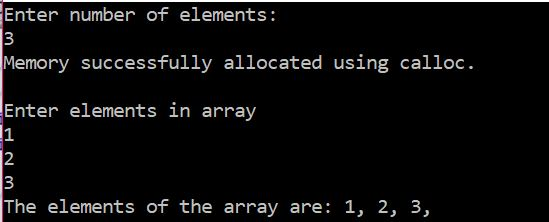### When to use calloc()

• Allocating memory to contain a structure, where you want all the members initialised to zero.
• Allocating memory for an array of chars which you are later going to write some number of chars into, and then treat as a NULL terminated string.
• Allocating memory for an array of pointers which you want initialised to NULL.

### realloc()

• It stands for re-allocation.
• When memory allocated previously is not sufficient i.e more memory is required you can use realloc to re-allocate memory dynamically.

Syntax :

``````rp = realloc(rp, newSize);
``````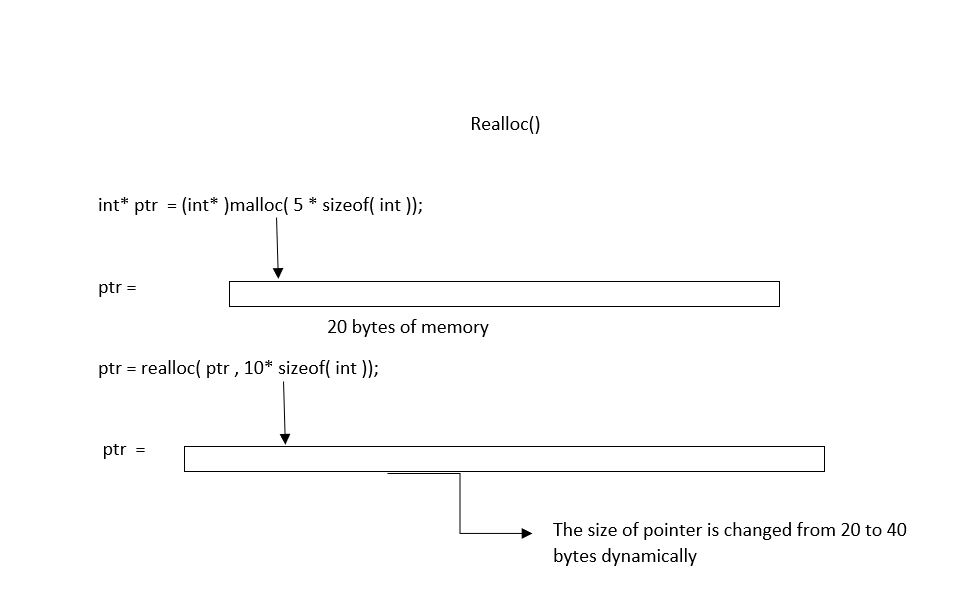Example :

``````mp = (char*) malloc(100 * sizeof(char));

mp = realloc(mp, 100 * sizeof(char)); // new memory allocated
``````

### Pseudocode

``````#include <stdio.h>
#include <stdlib.h>

int main()
{

// This pointer will hold the
// base address of the block created
int* ptr;
int n, i, sum = 0,m;

// Get the number of elements for the array

printf("Enter number of elements: \n");
scanf("%d",&n);

// Dynamically allocate memory using calloc()
ptr = (int*)calloc(n, sizeof(int));

// Check if the memory has been successfully
// allocated by malloc or not
if (ptr == NULL) {
printf("Memory not allocated.\n");
exit(0);
}
else {

// Memory has been successfully allocated
printf("Memory successfully allocated using calloc.\n");

// Get the elements of the array
printf("Enter elements in array :");
// Get the new elements of the array
for (i = 0; i < n; ++i) {
scanf("%d",&ptr[i]);
}

// Print the elements of the array
printf("The elements of the array are: ");
for (i = 0; i < n; ++i) {
printf("%d, ", ptr[i]);
}

// Get the new size for the array

printf("\n\nEnter the new size of the array: \n");
scanf("%d",&m);

// Dynamically re-allocate memory using realloc()
ptr = realloc(ptr, m* sizeof(int));

// Memory has been successfully allocated
printf("Memory successfully re-allocated using realloc.\n");
printf("Enter elements in array :");
// Get the new elements of the array
for (i = n; i < m; ++i) {
scanf("%d",&ptr[i]);
}

// Print the elements of the array
printf("The elements of the array are: ");
for (i = 0; i < n; ++i) {
printf("%d, ", ptr[i]);
}

free(ptr);
}

return 0;
}
``````

Output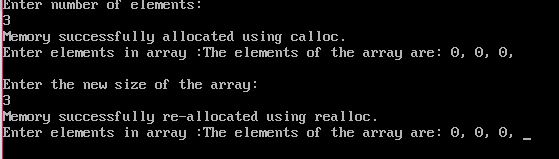### When to use realloc()

• When you want to resize your memory allocation.

### Difference between malloc() and calloc()

Initialization: malloc() allocates memory block of given size (in bytes) and returns a pointer to the beginning of the block. malloc() doesn’t initialize the allocated memory. If we try to acess the content of memory block then we’ll get garbage values.

``````void * malloc( size_t size );
``````

calloc() allocates the memory and also initializes the allocates memory block to zero. If we try to access the content of these blocks then we’ll get 0.

``````void * calloc( size_t num, size_t size );
``````

Number of arguments: Unlike malloc(), calloc() takes two arguments:

1. Number of blocks to be allocated.
2. Size of each block.

Return Value: After successfull allocation in malloc() and calloc(), a pointer to the block of memory is returned otherwise NULL value is returned which indicates the failure of allocation.

### Question

Consider the following code:

``````#include<stdio.h>

int main()
{
void *ptr;
ptr = (char *)calloc(10,sizeof(char));
*ptr = 'A';
printf("%c\n",*ptr);
return 0;
}
``````

#### In the above code, what will be the output?

Compilation error
A
65